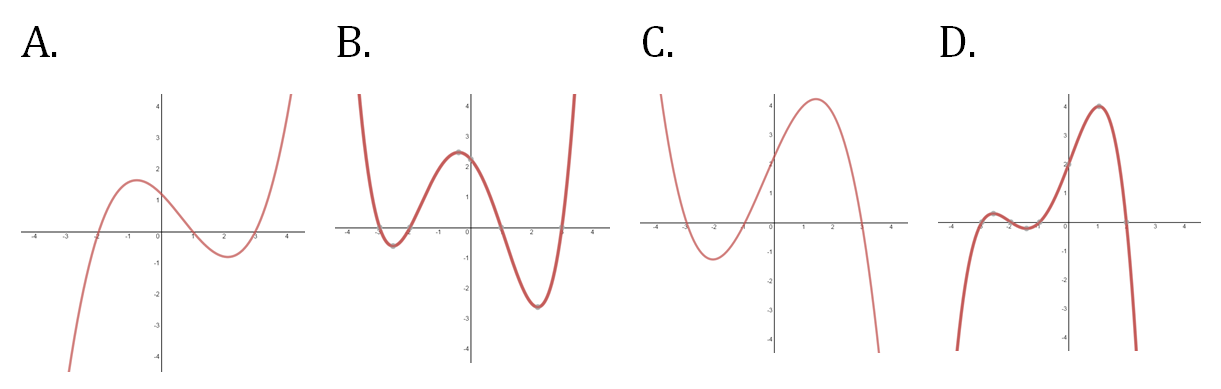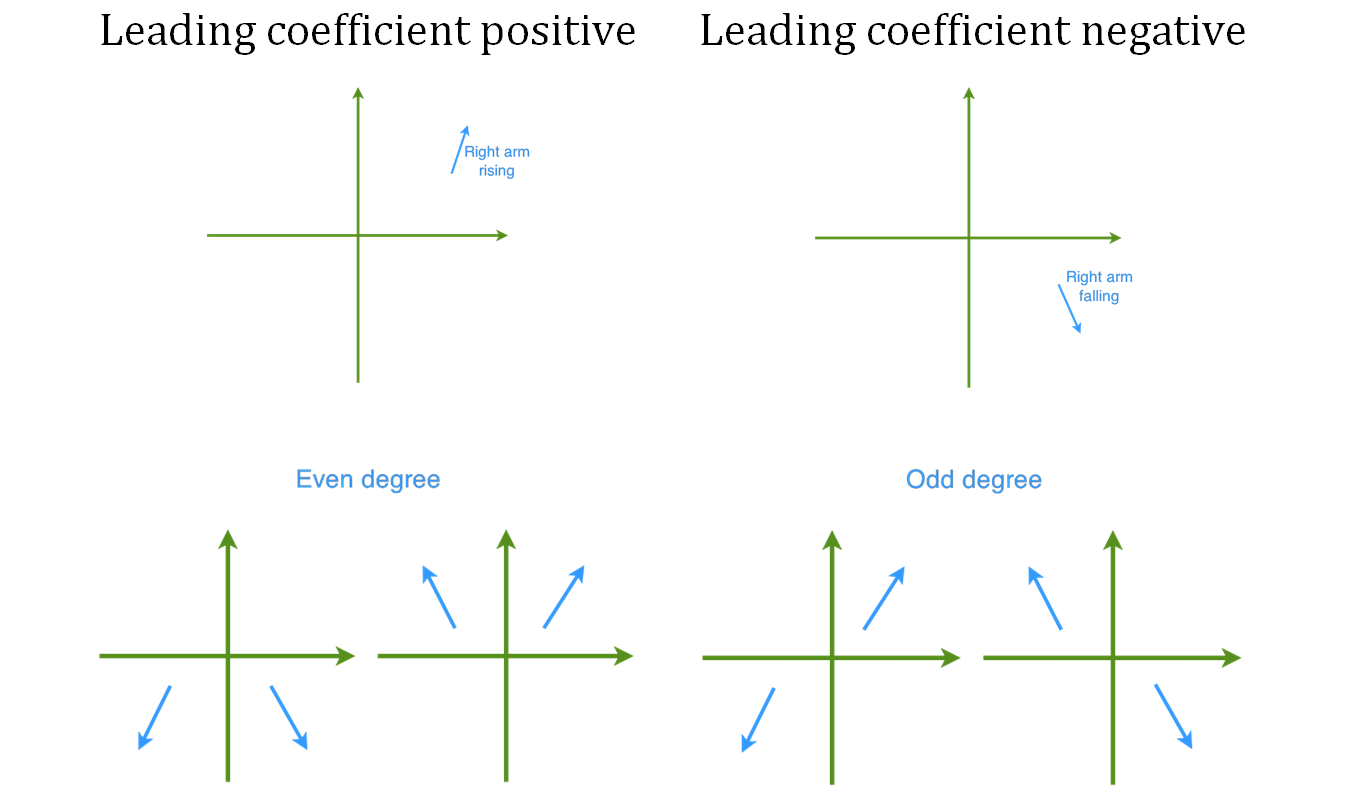# Characteristics of polynomial graphs#### Everything You Need in One Place

Homework problems? Exam preparation? Trying to grasp a concept or just brushing up the basics? Our extensive help & practice library have got you covered.#### Learn and Practice With Ease

Our proven video lessons ease you through problems quickly, and you get tonnes of friendly practice on questions that trip students up on tests and finals.#### Instant and Unlimited Help

Our personalized learning platform enables you to instantly find the exact walkthrough to your specific type of question. Activate unlimited help now!##### Examples
###### Lessons
1. Investigating the Graphs of Polynomial Functions
Recognize the characteristics of polynomial graphs from the following quadratic and cubic functions:
i)
$P\left( x \right) = {x^2} - 3x - 10$
ii)
$P\left( x \right) = - {x^2} + 3x + 10$
iii)
$P\left( x \right) = {x^3} - 3{x^2} - 10x$
iv)
$P\left( x \right) = - {x^3} + 3{x^2} + 10x$
1. Graphing Polynomials
Sketch the following polynomials:
1. $P(x)=(x-3)(x-1)(x+2)(x+5)$
2. $P(x)=-(x-5)(x-2)(x+1)(x+4)(x+6)$
3. $P(x)=-x^2-2x+8$
4. $P(x)=x^3-8x^2+15x$
2. Identifying Polynomial Graphs
Which of the following could be the graph of a quadratic function with a negative leading coefficient?###### Topic Notes